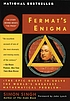Classify

Summary

Title:
Fermat's enigma : the quest to solve the world's greatest mathematical problem
Author:
Singh, Simon
Formats:

Editions:
30
Total Holdings:
2295
OCLC Work Id:
611345
http://classify.oclc.org/classify2/ClassifyDemo?owi=611345
Most Frequent 512.74 2272 Web Dewey
Edition: 22 512.74 42
Most Frequent QA244 2166 ClassWebFermat's last theorem 2262 922971
Wiles, Andrew 1734 391991Name VIAF ID
Singh, Simon 73996990

Editions

Displaying 1 to 25 of 30
Title and AuthorLanguageFormatHoldings TagClass NumberFermat's enigma : the quest to solve the world's greatest mathematical problemby Singh, Simoneng 1550050QA244
082512.74

(DDC 21)Fermat's enigma : the epic quest to solve the world's greatest mathematical problemby Singh, Simoneng 495050QA244
082512.74

(DDC 21)Fermat's enigma : the epic quest to solve the world's greatest mathematical problemby Singh, Simoneng 99082512.74

(DDC 21)Fermat's enigma : the quest to solve the world's greatest mathematical problemby Singh, Simoneng 26082512.74

(DDC 21)

050QA244Fermat's enigma : the epic quest to solve the world's greatest mathematical problemby Singh, Simoneng 22082512.74

(DDC 22)

050QA244Fermat's enigma : the quest to solve the world's greatest mathematical problemby Singh, Simoneng 19050QA244
082512.74

(DDC 21)Fermat's enigma : the epic quest to solve the world's greatest mathematical problemby Singh, Simoneng 15050QA244
082512.74

(DDC 22)Fermat's enigma : the quest to solve the world's greatest mathematical problemby Singh, Simoneng 14082512.74

(DDC 21)

050QA244Fermat's enigma : the epic quest to solve the world's greatest mathematical problemby Singh, Simoneng 9082512.74

(DDC 21)

050QA244Fermat's enigma : the quest to solve the world's greatest mathematical problemby Singh, Simoneng 8050QA244
082512.74

(DDC 21)Fermat's enigma : the epic quest to solve the world's greatest mathematical problemby Singh, Simoneng 6Fermat's enigma : the epic quest to solve the world's greatest mathematical problemby Singh, Simoneng 5082512.74

(DDC 22)Fermat's enigma : the epic quest to solve the world's greatest mathematical problemby Singh, Simoneng 4082512.74

(DDC 21)

050QA244Fermat's enigma : the epic quest to solve the world's greatest mathematical problemby Singh, Simoneng 3Fermatś enigma : the epic quest to solve the worldś greatest mathematical problemeng 3050QA244
082512.74

(DDC 21)Fermat's enigma : the epic quest to solve the world's greatest mathematical problemby Singh, Simoneng 2082512

(DDC 21)Fermat's enigma : the epic quest to solve the world's greatest mathematical problemby Singh, Simoneng 2Fermat's enigma : the epic quest to solve the world's greatest mathematical problemby Singh, Simoneng 1082512

(DDC 21)Fermat's enigma : the epic quest to solve the world's greatest mathematical problemby Singh, Simoneng 1082512.74

(DDC 21)Fermat's enigma : the epic quest to solve the world's greatest mathematical problemby Singh, Simoneng 1Fermat's enigma : the epic quest to solve the world's greatest mathematical problemby Singh, Simoneng 1Fermats̀ enigma : the epic quest to solve the Worlds̀ Greatest mathematical problemby Singh, Simoneng 1Fermat's enigma : the quest to solve the world's greatest mathematical problemby Singh, Simoneng 1082512.74

(DDC 21)Fermat's enigma : the epic quest to solve the world's greatest mathematical problemby Singh, Simoneng 1Fermat's enigma : the epic quest to solve the world's greatest mathematical problemby Singh, Simoneng 1
Displaying 1 to 25 of 30
.